## Main

In superconducting circuits, each electrical element such as an inductor, a capacitor or the Josephson element can add a degree of freedom. In the case of small circuits, by applying Devoret’s circuit theory20, a complete analytical description that takes into account all degrees of freedom can be obtained. However, when the circuits contain an increasing number of elements, as for example Josephson junction chains, even numerical solutions of the problem become difficult to obtain when taking into account all degrees of freedom. Nevertheless, our measurements demonstrate that the ground state of a phase-biased Josephson junction chain (see Fig. 1a) can be described by a single degree of freedom. Although the chain is a multidimensional object, the effect of quantum phase slips can be described by a single variable, m, that counts the number of phase slips in the chain.Figure 1: Graphic representations describing the effect of phase slips in a six-junction chain, the resulting chain’s energy and supercurrent and the measurement principle.

We start by giving a short introduction on the low-energy properties of a Josephson junction chain analysed in terms of quantum phase slips8. Let us consider the Josephson junction chain shown in Fig. 1a. The chain contains N junctions and is biased with a phase γ. We denote EJ the Josephson energy of a single junction and EC=e2/2C its charging energy. Here we consider EJEC. Let Qi be the charge on each junction and θi the phase difference. In the nearest-neighbour-capacitance limit, the Hamiltonian can be written as: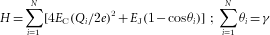Ignoring the charging energy for the moment, in the classical ground state the phase bias γ is equally distributed on the N junctions: θi=γ/N, as illustrated by the solid line in Fig. 1b. The resulting Josephson energy hence reads E0=EJγ2/2N and the chain is equivalent to a large inductance. If a phase slip occurs on one of the junctions, say the jth junction, then θjθj+2π. The constraint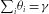would be violated after such a phase-slip event if the phases across all other junctions do not adjust. Therefore, the phase difference θi over all other junctions changes a little from γ/N to (γ−2π)/N to accommodate the bias constraint (see the dashed line in Fig. 1b). A phase slip on a single junction leads to a collective response of all junctions. Consequently, the Josephson energy of the entire chain changes from E0=EJγ2/2N to Em=EJ(γ−2πm)2/2N after m phase slips. The classical ground state energy of the chain consists of shifted parabolae that correspond respectively to a fixed number m of phase slips (see Fig. 1c). For the special values γ=π(2m+1), the energies Em and Em+1 are degenerate.

Taking now into account the finite charging energy EC, quantum phase slips can lift the degeneracy at the points γ=π(2m+1). In the limit of rare phase slips, that is, EJEC, the hopping element for the quantum phase slip can be approximated by21,22: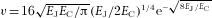. As a phase slip can take place on any of the N junctions, the hopping term between the two states |m〉 and |m+1〉 is given by N v. Therefore, using a tight-binding approximation, the total Hamiltonian for the chain is given by: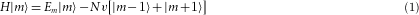Figure 1c shows the numerical calculation of the two lowest eigenenergies of the Hamiltonian (1) for three different ratios EJ/EC=20, 3 and 1.3 in the case of a six-junction chain. Figure 1d shows the corresponding current–phase relation of the chain in the ground state. The chain’s supercurrent is obtained by the calculation of the derivative of the ground-state energy Eg: iS=(2e/)( Eg/ γ). For large values of EJ/EC, quantum phase fluctuations are very small (v0) and the current–phase relation has a sawtooth-like dependence with a critical current that is approximatively N/π times smaller than that of a single junction of the chain. We call this regime the ‘classical’ phase-slip regime. When quantum phase fluctuations increase, that is, EJ/EC decreases, the current–phase relation becomes sinusoidal and the critical current becomes exponentially suppressed with N and EJ/EC (ref. 8).

To measure the effect of quantum phase slips on the ground state of a Josephson junction chain, we have studied a chain of six junctions. Our measurement set-up and the junction parameters are presented in Fig. 2 and Table 1. Each junction in the chain is realized by a superconducting quantum interference device (SQUID) to enable tunable Josephson coupling EJ. In this way we can tune in situ the EJ/EC ratio by applying a uniform magnetic flux ΦS through all SQUIDs, and consequently we can control the strength of quantum phase fluctuations. For our measurements we placed this chain in a closed superconducting loop, threaded by the flux ΦC, containing an extra shunt Josephson junction that is used for the read-out of the chain state. The flux ΦC enables the control of the bias phase γ=ΦCδ over the chain.

We have measured the switching current of the entire Josephson junction circuit containing both the chain and the read-out junction. The switching current was determined from the switching probability at 50%. The switching probability as a function of bias current Ibias has a width of ≈20 nA. We apply typically 10,000 bias-current pulses of amplitude Ibias and measure the switching probability as the ratio between the number of switching events and the total number of pulses. The current pulses have a rise time of 8 μs and a total duration of 20 μs. The results of the switching-current measurements as a function of flux ΦC are shown in Fig. 3. From these switching-current measurements we deduce the effect of quantum phase slips on the ground state of the chain.Figure 3: Measured switching current (black diamonds) as a function of φC over the chain for three different EJ/EC ratios.

The measured switching current corresponds to the escape process out of the total potential energy Utot containing the contributions of the read-out junction and the chain: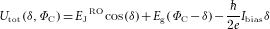Here Eg is the ground state of the six-SQUID chain calculated by solving the Hamiltonian (1). As EJROEg the main component in Utot is the potential of the current-biased read-out junction EJROcos(δ)−(/2e)Ibiasδ. Figure 1f shows the escape potential at constant bias current for three different flux values φC corresponding to three different biasing phases γ over the chain. Let us point out that the position of the minimum of the potential Utot is in good approximation independent of the value of the flux φC. Therefore, the bias phase difference γ over the chain depends only on the flux φC. As a consequence, the φC dependence of the measured switching current results from the γ dependence of the chain’s ground state.

The escape from the potential Utot occurs by means of macroscopic quantum tunnelling (MQT). The MQT rate for an arbitrary potential can be calculated in the limit of weak tunnelling using the dilute instanton-gas approximation23. Within this model, the escape rate Γ out of the washboard potential Utot(γ) reads24: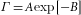where A and B are given by:We have denoted by σ the width of the barrier and by x the phase coordinate measured from the minimum of the washboard potential. The plasma frequency is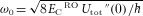, where ECRO is the charging energy of the read-out junction.

Knowing the escape rate Γ, we can calculate the switching probability: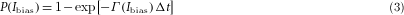The results of numerical calculations and the experimental data are shown in Fig. 3. The theory fits very well both in amplitude and shape the oscillations of the measured switching current. Let us point out that we have used the nominal values for EJ and EC calculated from the characteristics of the sample indicated in Table 1. The normal-state resistance for a single chain junction has been deduced from the measured normal-state resistance of the read-out junction by considering the size ratio between the two. We evaluate the precision of the determination of EJ and EC to be in the range of ±10%. This error bar on EJ and EC yields an uncertainty of ±15% for the phase-slip amplitude N v. The eventual presence of junction inhomogeneity or an important effect of background charges would imply a significantly larger decrease of the phase-slip amplitude8. The good agreement between theory and experiment confirms the homogeneity of our junctions. It excludes a significant contribution of background charges in the overall shape of the switching curve and demonstrates the collective nature of the phase-slip events.

From the measurements in Fig. 3, we define the switching-current amplitude ΔISW as half of the peak-to-peak variation of the switching current with the flux ΦC. Figure 4 shows the measured ΔISW and the corresponding theoretical calculations as a function of EJ/EC. For each measurement, EJ has been calculated using the flux dependence of the SQUID’s Josephson coupling: EJ(ΦS)=(/2e)i0(ΦS) with i0(ΦS)=ICSQcos(πΦS/Φ0). To distinguish between the suppression of the switching current that is due to quantum phase fluctuations and the one that is simply due to the well-known cancellation of the SQUID’s critical current as a function of flux, we plot the switching-current amplitude divided by the critical current of a single SQUID i0. We see that the measured switching-current amplitude follows very well the predicted theoretical suppression of the switching-current oscillations in the presence of quantum phase fluctuations. From our measurements we can also deduce the strength of the quantum phase-slip amplitude. With decreasing EJ/EC ratio from 3 to 1 the quantum phase-slip amplitude increases from 0.8 to 2.7 GHz. In addition, in Fig. 4 we have plotted for comparison the calculation for the switching-current amplitude in the case when quantum phase fluctuations would be negligibly small: v0. As expected, we get a practically flat dependence on EJ/EC.Figure 4: Comparison between the measured and the calculated switching-current amplitude as a function of the EJ/EC ratio.

Further on, the upper x axis of Fig. 4 shows the ratio EJ/kBT of the Josephson energy with respect to the thermal energy at T=50 mK. As EJkBT, thermal fluctuations are excluded to explain the suppression of the switching current with decreasing EJ/EC. Further measurements (not shown here) reveal a constant switching-current amplitude and width of the switching distribution up to a temperature of T=100 mK.

We present a detailed experimental characterization of the effect of quantum phase slips on the ground state of a Josephson junction chain. These phase slips are the result of fluctuations induced by the finite charging energy of each Josephson junction in the chain. The experimental results can be fitted in very good agreement by considering a simple tight-binding model for the phase slips8. Our measurements also show that a Josephson junction chain under phase-bias constraint can behave in a collective way very similar to a single macroscopic quantum object.

These results open the way for the use of quantum phase slips in Josephson junction networks for the implementation of a new current standard, the observation of Bloch oscillations19, the fabrication of topologically protected qubits25 and the design of new superconducting circuit elements.

## Methods

The circuit was fabricated on a Si/SiO2 substrate and the Al/AlOx/Al junctions were obtained using standard shadow evaporation techniques. The aluminium oxide was obtained by natural oxidation in a controlled O2 atmosphere. Room-temperature measurements on an ensemble of 100 junctions revealed a variance of 4% of the normal-state resistance of the junctions.

The sample was mounted in a closed copper block that was thermally connected to the cold plate of a dilution refrigerator at 50 mK. All lines were strongly filtered by low-pass filters at the cryostat entrance and by thermocoaxial cables and π filters at low temperatures.

The switching current ISW of the circuit is obtained by carrying out the following sequence. We use a series of M current steps of equal amplitude Ibias to bias the junction. We count the number of transitions to the voltage state MSW and thus obtain the value of the switching probability PSW=MSW/M corresponding to the applied Ibias. By sweeping the Ibias amplitude and repeating the above sequence, we measure a complete switching histogram, PSW versus Ibias. The PSW=50% bias current is called the switching current of the circuit, ISW.

The principle of the read-out scheme was first implemented by Vion et al. 26 and has also been used for the measurement of the ground state of superconducting atomic contacts27. The choice of the read-out junction critical current ICRO for an optimal measurement of iS is not straightforward. On the one hand one would like to have ICROiS, but on the other hand the width of the switching histograms w increases with ICRO and so do the statistical fluctuations resulting from finite ensemble size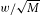. For reasonable measuring timescales, the number of current steps M is limited to values of about 104. If we want to measure supercurrents for the SQUID chain in the range of 1 nA, ICRO needs to be in the range of 100 nA. We have used a read-out junction with a critical current ICRO=330 nA that offers a good trade-off.

In our MQT analysis we neglect the effect of dissipation on the escape rate. Small dissipation can add a pre-factor in front of the exponential in the switching probability formula (3). However, this factor is independent of ΦC, so it will change only the offset value of ISW in Fig. 3, but not the shape nor the amplitude of the ISW oscillations.